## Equation-solving front page

### Quick description

There are several different kinds of equations, and techniques for solving them vary considerably. So this page consists mainly of links to pages about solving particular kinds of equations.

There are a few general principles, however.

### General discussion

If we have a number, there are many things we can do to it. For example, we can add 2 to it, or multiply it by 17, or square it. Or one can combine two such operations and obtain a number like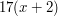. More ... ( This leads to a general class of questions of the following form: if I start withand do such-and-such to it and get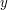, then what is? Here we think ofas given andas something that is calculated from. Equations arise when one reverses this question and asks instead the following: if I started withand did such-and-such to it, and the answer came out to be, then what was? This timeis given andis to be determined. To give a simple example, if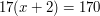, we can work out that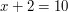and therefore that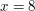. Questions of this kind do not have to be about numbers. For example, we could ask a question like this: I start with a function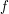, differentiate it, and end up with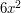; what was the original function? This is a simple differential equation, and the unknown object is the function. In this case, the equation does not fully determine, but we can at least say thatmust be of the form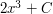for some constant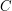. As this simple example already suggests, the number of different kinds of equation is more or less the same as the number of different kinds of processes we like to do to mathematical objects. Since there are many kinds of processes, there are many kinds of equations. Further below is a list of different kinds, with brief explanations of what they are and links to articles or navigation pages about them. However, some techniques for solving equations are very general, so those are linked first.)

### Generic equation-solving techniques

Transform equation to reach a solved pattern Brief summary ( Make logically equivalent rearrangements of the equation until it, or the "hard" part of it, matches a pattern you already know how to deal with. )

### Articles about different kinds of equations

How to solve linear equations in one variable Brief summary ( These are equations like. This equation is called linear because the graph of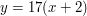is a straight line. )

How to solve linear equations in two variables Brief summary ( Things get slightly more complicated if you have two unknowns, and two equations to help you to determine them. For example, the equations could be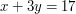and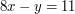. However, there are various systematic ways of solving such pairs of equations and determiningand. )

How to solve linear equations in many variables Brief summary ( The methods used to solve linear equations in two variables can be generalized to any number of variables. At this point it becomes fruitful to think of the unknown as a vector rather than as a sequence of many individual variables. )

How to solve quadratic equations Brief summary ( A quadratic equation is something like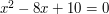, which involves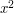as well as. There are several techniques for solving them: which is best varies from example to example. )

How to solve cubic and quartic equations Brief summary ( These are like quadratic equations, but now they also involve cubes and fourth powers, respectively. For instance, the equation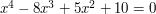is a quartic equation. Cubic and quartic equations can be solved systematically, but they are much harder than quadratic equations. )

What makes some equations so much easier to solve than others? Brief summary ( There are several answers one might give to this question but here is one: if you can work something out on your calculator without using any memory, then the resulting equation will be easy to solve. A few examples will make clear what this means. )

How to solve polynomial equations in several variables

How to solve linear Diophantine equations

How to solve quadratic Diophantine equations

Diophantine equations front page

Differential equations front page Brief summary ( Differential equations are equations where the unknown involves a function (or more than one function) and the equation involves not just the function but also some of its derivatives. If the function is of more than one variable, then these may be partial derivatives. There are many importantly different kinds of differential equation. This page gives some initial guidance. )

How to solve functional equations